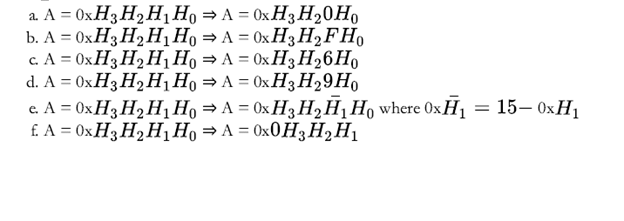# Homework 1 Solved

20.00 \$ 10.00 \$

Category:

You'll get a: . ` zip` file solution link after purchase

## Description

1. (60 points total, 10 points each) Determine the result in both decimal and binary format and C and V flags

Of the following calculations for a 5-bit system using the C and V flag convention of an ARM processor:

a. (-16) + (-16)

b. 14 – (-16)

c. 14-16

d. 15- (-6)

e. 15+ (-6)

f. 14+ (-16)

1. (30 points total, 5 points each) Write the C code to perform the following operations assuming A is a uint16 t variable: